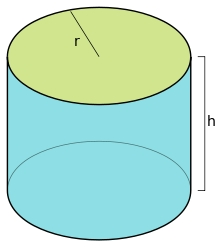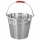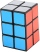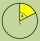# Ratio of volumes

If the heights of two cylindrical drums are in the ratio 7:8 and their base radii are in the ratio 4:3. What is the ratio of their volumes?

p =  14:9

### Step-by-step explanation:Did you find an error or inaccuracy? Feel free to write us. Thank you!Tips to related online calculators
Need help to calculate sum, simplify or multiply fractions? Try our fraction calculator.
Check out our ratio calculator.
Tip: Our volume units converter will help you with the conversion of volume units.

#### You need to know the following knowledge to solve this word math problem:

We encourage you to watch this tutorial video on this math problem:

## Related math problems and questions:

• Volume and surfaceCalculate the volume and surface area of the cylinder when the cylinder height and base diameter is in a ratio of 3:4 and the area of the cylinder jacket is 24 dm2.
• Top-open tankThe top-open tank has the shape of a truncated rotating cone, which stands on a smaller base. The tank's volume is 465 m3, the radii of the bases are 4 m and 3 m. Find the depth of the tank.
• Interior anglesCalculate the interior angles of a triangle that are in the ratio 2: 3: 4.
• Perimeter of baseThe circumference of the base of the rotating cylinder is same as its height. What is the diameter and height of this cylinder with volume 1 liter?
• Cuboid edgesThe lengths of the cuboid edges are in the ratio 2: 3: 4. Find their length if you know that the surface of the cuboid is 468 m2.
• Three segmentsThe circle is divided into 3 segments. Segment A occupies 1/4 of the area. Segment B occupies 1/3 of the area. What part is occupied by section C? In what proportion are areas A: B: C?
• Cylinder - hThe cylinder volume is 140 cm3. The base radius is 7 cm. Calculate the height of the cylinder.
• ContainerThe container has a cylindrical shape the base diameter 0.8 m and the area of the base is equal to the area of the wall. How many liters of water can we pour into the container?
• DiameterWhat is the inside diameter of the cylinder container and if half a liter of water reaches a height 15 cm?
• Cuboid - edgesThe cuboid has dimensions in ratio 4: 3: 5, the shortest edge is 12 cm long. Find: (A) the lengths of the remaining edges, (B) the surface of the cuboid, (C) the volume of the cuboid
• Cylinder and its circumferenceIf the height of a cylinder is 4 times its circumference c, what is the volume of the cylinder in terms of its circumference, c?
• Tank and waterWe poured a cylindrical tank with 3.5 liters of water. If the tank base diameter is 3 dm, how height is the water level in?
• Cylindrical tankCylindrical tank holds 600hl water and is deep 2.5 m. Calculate the diameter of the cylinder.
• Sphere in coneA sphere is inscribed in the cone (the intersection of their boundaries consists of a circle and one point). The ratio of the surface of the ball and the contents of the base is 4: 3. A plane passing through the axis of a cone cuts the cone in an isoscele
• Cuboid - ratioFind the volume of a block whose dimensions are in the ratio 2: 3: 4 and the surface is 117 dm2.
• Cylindrical tank9.6 hl of water is poured into a cylindrical tank with a bottom diameter of 1.2 m. What height in centimeters does the water reach?
• The cylinder 2Find the volume and the lateral area of a cylinder of height 12 inches and a base radius of 4 inches.